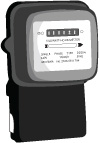# Calculating the Electricity Tariff

The amount of electricity consumed by an electrical appliance (energy consumption) is calculated by multiplying its power by the duration of use. Energy consumption is usually measured in kilo-Watt-hours (kWh), which is commonly expressed as “one unit of electricity”.

For example, if a 1,500W electric heater is used for 2 hours, its energy consumption is

1,500W x 2h = 3,000Wh = 3 kWh

The electricity tariff of most households is calculated on a monthly or bimonthly basis. The total energy consumption of a household is the total amount of energy consumed by all appliances which are or have been in operation. The tariff is calculated by multiplying the total energy consumption by the price per unit of electricity (kWh).

Therefore, the more appliances a household has, and/or the higher the power rating or the longer duration of use, the more expensive the tariff is.The main function of an electric meter is to measure the energy consumption for calculation of electricity tariff.
4 December 2020